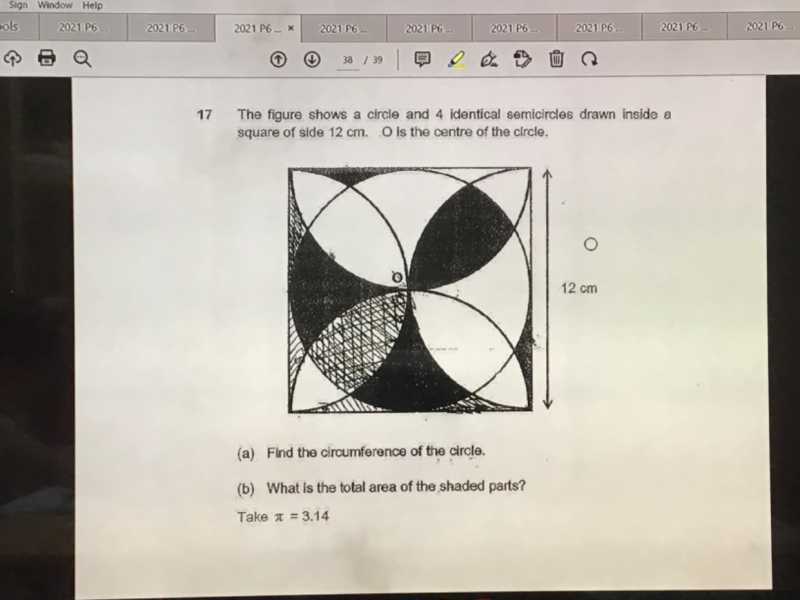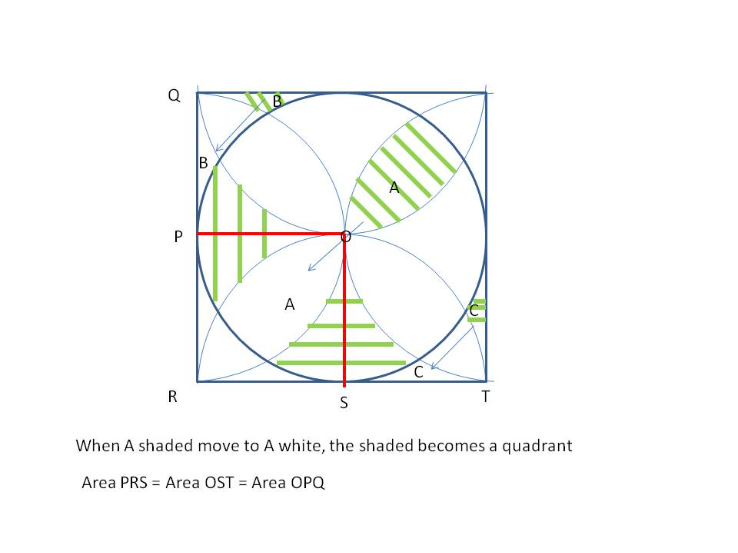# QuestionPls help to solve (b), thank you(b)  Move shaded A to white A

Move shaded B to white B

Move shaded C to white C

So new shaded region is OQPRST made of Area OPS, Area OST and Area OPQ

Area OPS is a quadrant,  so Area =  πr2 / 4  =  (3.14 x 6 x 6) / 4 = 28.26

Area PRS = (Area of Square – Area of circle) / 4 =  [144 – (3.14 x 6 x 6)] / 4 = 7.74

Area PRS = Area OST = Area OPQ

Hence Area of shaded parts = 28.26 + 2(7.74) = 43.74 cm2